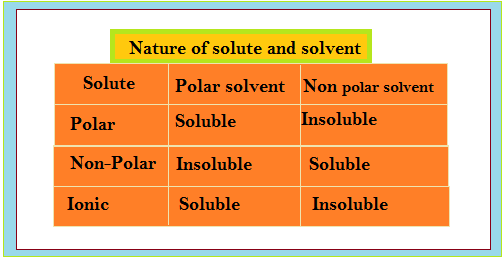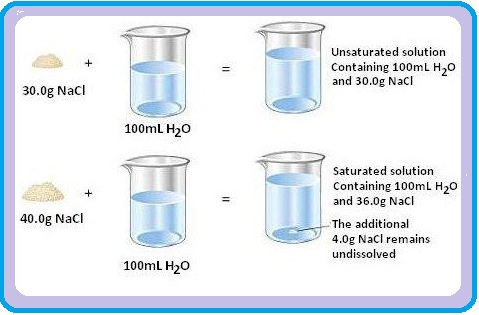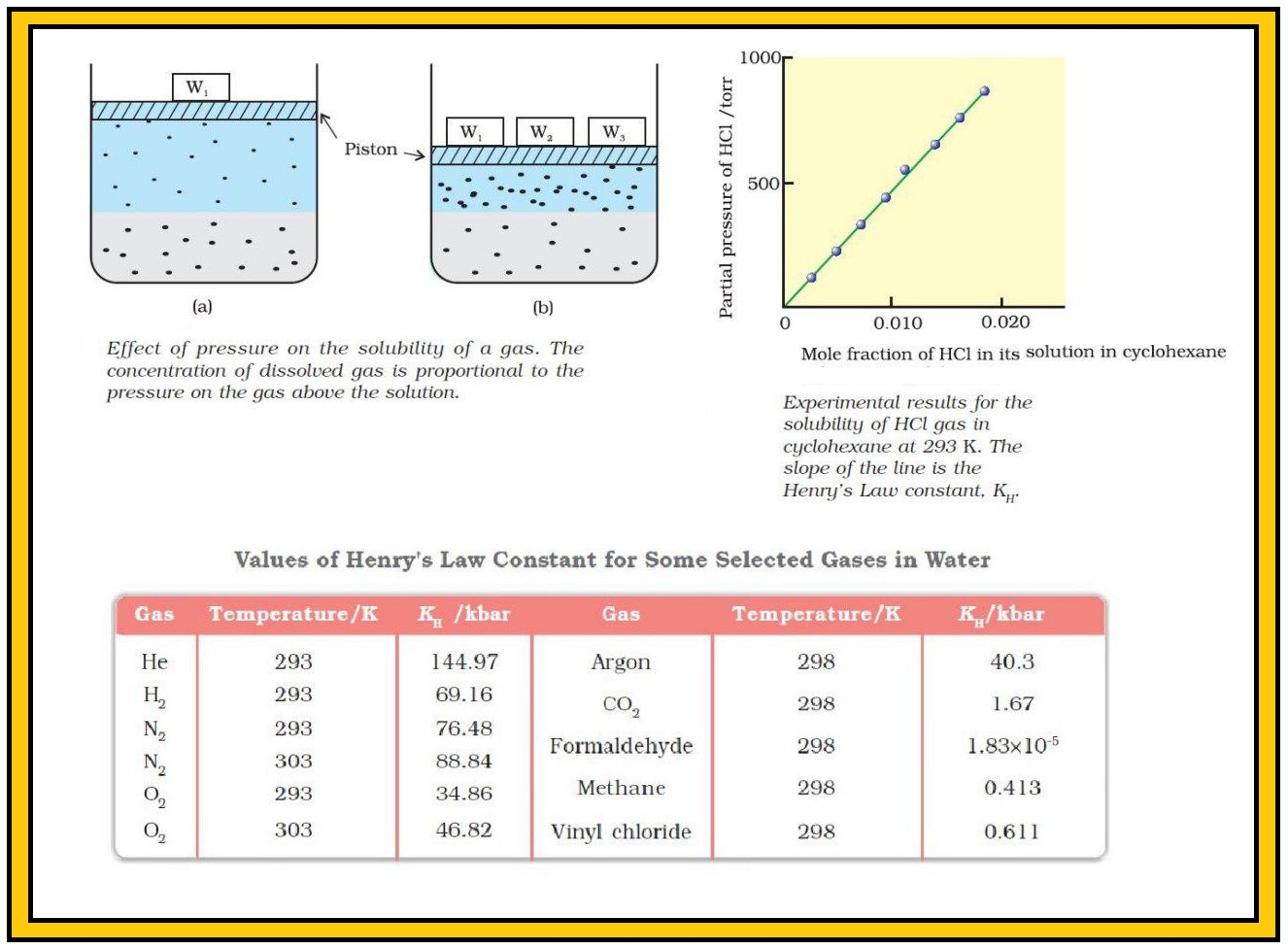Chemistry Solubility, Henry's Law and it's Applications
Click for Only Video

### Topics Covered :

● Solubility
● Solubility of a Solid in a Liquid
● Solubility of a Gas in a Liquid
● Henry's Law
● Applications of Henry's Law
● Effect of Temperature on Solubility of Gases

### Solubility :color{green}("Definition") : It's the maximum amount of a substance that can be dissolved in a specified amount of solvent.

=> It depends upon the nature of solute and solvent as well as temperature and pressure.

### Solubility of a Solid in a Liquid :Every solid does not dissolve in a given liquid.

=> NaCl and sugar dissolve readily in water. Naphthalene and anthracene do not.

=> Naphthalene and anthracene dissolve readily in benzene but sodium chloride and sugar do not.

=> It is observed that polar solutes dissolve in polar solvents and non polar solutes in non polar solvents.

=> In general, a solute dissolves in a solvent if the intermolecular interactions are similar in the two or we may say like dissolves like.

=> color{green}("Dissolution") : When a solid solute is added to the solvent, some solute dissolves and its concentration increases in solution. This process is known as dissolution.

=> color{Green}("Crystallisation") : Some solute particles in solution collide with the solid solute particles and get separated out of solution. This process is known as crystallisation.

=> When two processes occur at the same rate, number of solute particles going into solution will be equal to the solute particles separating out and a state of dynamic equilibrium is reached.

color{green}("Solute + Solvent ⇋ Solution") .....................1

At this stage the concentration of solute in solution will remain constant under the given conditions, i.e., temperature and pressure.

=> Similar process is followed when gases are dissolved in liquid solvents.

=> color{green}("Saturated Solution") : A solution in which no more solute can be dissolved at the same temperature and pressure is called a saturated solution.

=> color{green}("Unsaturated Solution") : An unsaturated solution is one in which more solute can be dissolved at the same temperature.

=> The solution which is in dynamic equilibrium with undissolved solute is the saturated solution and contains the maximum amount of solute dissolved in a given amount of solvent.

=> Thus, the concentration of solute in such a solution is its solubility.

=> Solubility of one substance into another depends on the nature of the substances. In addition to these variables, two other parameters, i.e., temperature and pressure also control this phenomenon.

color{green}("Effect of temperature") : Equation 1 must follow the Le Chateliers Principle.

=> If dissolution process is endothermic (color{green}(Δ_text(sol) H > 0)), the solubility should increase with rise in temperature

=> If it is exothermic (color{green}(Δ_text(sol) H < 0)) the solubility should decrease.

color{green}("Effect of pressure") :

=> Pressure does not have so much effect on solubility of solids in liquids.

=> It is so because solids and liquids are highly incompressible and practically remain unaffected by changes in pressure.

### Solubility of a Gas in a Liquid :=> Oxygen dissolves in water to a small extent. This dissolved oxygen sustains all aquatic life.

=> On the other hand, hydrogen chloride gas (HCl) is highly soluble in water.

=> Solubility of gases in liquids is greatly affected by pressure and temperature.

=> The solubility of gases increase with increase of pressure.

color{green}("Explanation") : For solution of gases in a solvent, consider a system as shown above. The lower part is solution and the upper part is gaseous system at pressure p and temperature T. In a state of dynamic equilibrium, i.e., under these conditions rate of gaseous particles entering and leaving the solution phase is the same. Now increase the pressure by compressing the gas to a smaller volume. This will increase the number of gaseous particles per unit volume over the solution and also the rate at which the gaseous particles are striking the surface of solution to enter it. So, the solubility of the gas will increase until a new equilibrium is reached resulting in an increase in the pressure of a gas above the solution and thus its solubility increases.

color{green}("Henry's Law") :

=> Henry gave a quantitative relation between pressure and solubility of a gas in a solvent which is known as Henry’s law.

color{green}("Statement") : At a constant temperature, the solubility of a gas in a liquid is directly proportional to the pressure of the gas.

=> Dalton, at the same time, also concluded independently that the solubility of a gas in a liquid solution is a function of partial pressure of the gas.

=> If mole fraction of a gas in the solution is used as a measure of its solubility, then mole fraction of gas in the solution is proportional to the partial pressure of the gas over the solution.

=> The most commonly used form of Henry’s law states that “the partial pressure of the gas in vapour phase (p) is proportional to the mole fraction of the gas (x) in the solution” and is expressed as :

color{green}(p = K_H x) ............2

Here color{geen}(K_H) is the Henry’s law constant.

=> A graph between partial pressure of the gas versus mole fraction of the gas in solution, then we get a plot of as shown above.

=> Different gases have different K_H values at the same temperature (Table). This shows that K_H is a function of the nature of the gas.

=> Higher the value of K_H at a given pressure, the lower is the solubility of the gas in the liquid.

=> K_H values for both N_2 and O_2 increase with increase of temperature showing that the solubility of gases increases with decrease of temperature (Table).

=> Due to this reason that aquatic species are more comfortable in cold waters rather than in warm waters.
Q 2965380265If N_2 gas is bubbled through water at 293 K, how many millimoles of N_2 gas would dissolve in 1 litre of water. Assume that N_2 exerts a partial pressure of 0.987 bar. Given that Henry’s law constant for N_2 at 293 K is 76.48 k bar.
NCERTSolution:

The solubility of gas is related to the mole fraction in aqueous solution. The mole fraction of the gas in the solution is calculated by applying Henry’s law. Thus:

x (text(Nitrogen)) = (p (text[nitrogen]))/K_H = (0.987 text(bar))/(76,480 text(bar)) = 1.29 × 10^-5

As 1 litre of water contains 55.5 mol of it, therefore if n represents number of moles of N_2 in solution,

x (text(Nitrogen)) = (n \ \ mol)/(n \ \ mol + 55.5 \ \ mol) = n/55.5 = 1.29 xx 10^-5

(n in denominator is neglected as it is < < 55.5)

Thus n = 1.29 × 10–5 xx 55.5 \ mol = 7.16 xx 10^–4 \ mol

= (7.16xx10^- 4 \ mol xx 1000 \ mol)/(1 \ mol) = 0.716 mmol

### Applications of Henry's Law :Henry’s law has many applications in industry and explains some biological phenomena. These are :

=> For increasing the solubility of CO_2 in soft drinks and soda water, the bottle is sealed under high pressure.

=> Increased pressure increases the solubility of atmospheric gases in blood. When the Scuba divers come towards surface, the pressure gradually decreases. This releases the dissolved gases and forms bubbles of nitrogen in the blood. This blocks capillaries and creates a medical condition known as bends, which are painful and dangerous to life. To avoid bends and the toxic effects of high concentrations of nitrogen in the blood, scuba divers use tanks filled with air diluted with helium (11.7% helium, 56.2% nitrogen and 32.1% oxygen).

=> At high altitudes the partial pressure of oxygen is less than that at the ground level. Therefore, there is low concentrations of oxygen in the blood and tissues of people living at high altitudes or climbers. Low blood oxygen makes climbers to become weak and unable to think clearly, symptoms of a condition known as anoxia.

### Effect of Temperature :=> Solubility of gases in liquids decreases with rise in temperature.

=> When dissolved, the gas molecules are present in liquid phase and the process of dissolution is similar to condensation and heat is evolved in this process. Dissolution process involves dynamic equilibrium and thus must follow Le Chatelier’s Principle.

=> As dissolution is an exothermic process, the solubility should decrease with increase of temperature.